# Sample Algebra I Lesson: How To Find Area & Volume

Objective:
At the end of this lesson you will be able to:

1. Find the area of a circle, rectangle, or square.
2. Find the volume of a rectangular prism.
3. Form an expression for the area of a combination of figures.
4. Find the area involving a combination of figures.

Prior Knowledge

• Multiplying Whole Numbers
• Multiplying Decimals
• Evaluating Exponents
• Evaluating Expressions and Formulas

### LESSON SUMMARY

In this lesson, we learn about the areas of circles, rectangles, and squares, and the volume of rectangular prisms. First, let’s look at the area of a square. The area of a figure measures its coverage in two dimensions. For example, the square below has an area of 4 square units.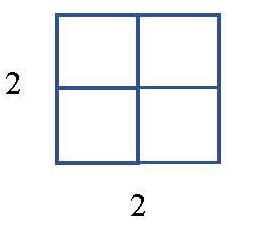The length of each side is 2 units, and the area of the square is 4 units, which is 2 x 2, or 2 squared. The area of a square with sides of length is A = s2, where A is the area and s is the length of each side.

Example: Alfred wants to create a garden in the shape of a square that is 20 feet long on each side. Find the area of the garden in square feet.

Solution: We want to find the area of a square with sides of length 20 feet. Substituting 20 for s in our formula, A = s2, we have A = (20)2, or A = 400. Since our units are in feet, our answer will be in square feet. The basketball court will have an area of 400 square feet, or 400 ft2.

Next, a rectangle has two dimensions, length and width, and the area is given by A = lw.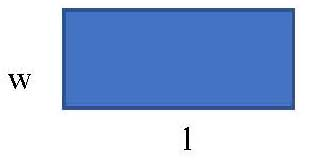Example: Suzanna wants to build a deck in the shape of a rectangle that is 20 feet long and 12 feet wide. Find the area of the deck in square feet.

Solution: We want to find the area of a rectangle with a length of 20 feet and a width of 12 feet. Substituting 20 for l and 12 for w into the formula, A = lw, we have A = (20)(12), or A = 240. Since our units are in feet, our answer will be in square feet. The deck will have an area of 240 square feet, or 240 ft2.

The area of a circle is given in terms of the symbol pi, π. We will use 3.14 for the value of π. The formula is A = πr2, where r is the radius of the circle.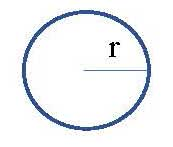Example: We want to find the area of a circle with a radius of 10 inches.

Solution: We can substitute 10 for r and 3.14 for π in the formula A = πr2, which gives us A = 3.14(10)2. 10 x 10 is 100, and 100 x 3.14 is 314, so the area of the circle is 314 square inches. This can also be written as 314 in2.

We can also find the area of a combination of figures. For example, we can find the area shaded below. The large rectangle has dimensions of 12 yards for length and 5 yards for width, while the small rectangle has dimension of 6 yards for length and 3 yards for width (which are not labelled in the picture).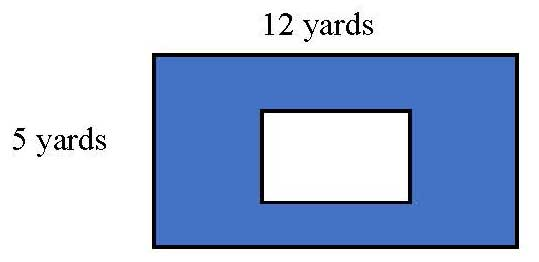Solution: To find the shaded area, we need to subtract the area of the small rectangle from the area of the large rectangle. Using the formula A = lw, the area of the large rectangle is A = (12)(5) = 60, and the area of the small rectangle is A = (6)(3) = 18. The shaded area will be 60 – 18 = 42, or 42 square yards. This can also be written as 42 yd2.

Next, let’s find an expression which represents the area of a combination of figures.

Example: Find the area shaded below. The large square has sides of length S, while the small square has sides of length s. We want to find an expression for the shaded area.Solution: To find the shaded area, we need to subtract the area of the small square from the area of the large square. The area of the large square is A = S2 and the area of the small rectangle is A = s2. The shaded area will be the difference, which is S2 – s2.

Now let’s look at volume, which is a measure involving three dimensions. We will find the volume of a rectangular prism, which has the shape of a box. The three dimensions of a rectangular prism, or box, are length, width, and height, and the area is found by multiplying these dimensions. The volume will be in units cubed, which we will call cubic units. The volume of a rectangular prism is given by V = lwh.

Example: A large classroom has the following dimensions: the length is 30 feet, the width is 25 feet, and the height is 10 feet. We want to find the volume of the room.

Solution: To find the volume, we can substitute the values of 30 for l, 25 for w, and 10 for h into the formula V = lwh. We have V = (30)(25)(10) = 7500, so the volume is 7500 cubic feet. This can also be written as 7500 ft3.

Examples

1. Find the area of a rectanle with a length of 3 meters and a width of 5 meters.

Solution: The area of a rectangle is given by A = lw, where l is the length and w is the width. Substituting the values of 3 for l and 5 for w into the formula A = lw gives us A = (3)(5), or A = 15. The area of the square is 15 square meters, or 15 m2.

2. An acre can be approximated as a square, with 210 feet on each side. Find the area of this square in terms of square feet, which will give the approximate square feet in an acre.

Solution: The area oa a square is A = s2, where s is the length of a side of the square. Substituting the value of 210 feet for s into the formula A = 2 gives us A = (210)2, or A = 44100. The area of the square is 44100 square feet, or 44100 ft2. This is the approximate area of an acre of land.

3. An above-ground swimming pool is formed in the shape of a circle, with a radius of 10 feet. Find the area of ground covered by the swimming pool in terms of square feet. Use 3.14 for π.

Solution: The area of a circle is A = π r2, where r is the radius of the circle. Substituting 3.14 for π and 10 for r into the formula gives us A = (3.14)(10)2. Simplifying, we have 102 = 10 x 10 = 100. So we have A = 3.14(100), or A = 314. The area of the ground covered by the swimming pool is 314 square feet, or 314 ft2.

4. Find the volume of a room that is 10 feet wide, 15 feet long, and 8 feet high? State your answers in terms of cubic feet.

Solution: The volume of a rectangular prism, which is the shape of this room, is given by V = lwh, where l is the length, w is the width, and h is the height. Substituting 15 for l, 10 for w, and 8 for h into the formula V = lwh, we have V = (15)(10)(8). Simplifying, we have 15 x 10 = 150, and then 150 x 8 = 1200. The volume of the room is 1200 cubic feet, or 1200 ft3.

5. Find the expression for the shaded area in the figure below.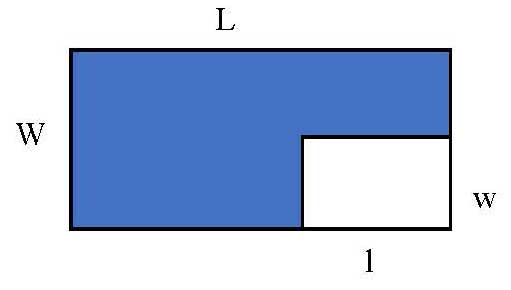Solution: The area of the shaded figure is the difference between the large rectangle with dimensions L and W, and the small rectangle with dimensions l and w. The area of the large rectangle is A = LW, while the area of the smaller rectangle is A = lw. The difference of the areas of the two rectangles would be given by the expression LW – lw.

Practice Problems

1. Find the area of a rectangle with a length of 4 inches and a width of 6 inches.
2. An quarter acre can be approximated as a square, with 105 feet on each side. Find the area of this square in terms of square feet, which will give the approximate square feet of a quarter acre.
3. An above-ground swimming pool is formed in the shape of a circle, with a radius of 20 feet. Find the area of ground covered by the swimming pool in terms of square feet. Use 3.14 for π.
4. Find the volume of a hallway that is 15 feet wide, 20 feet long, and 10 feet high?
5. Find the expression for the shaded area in the figure below.1. 24 square inches
2. 11025 square feet
3. 1256 square feet
4. 3000 cubic feet
5. 120 square meters

Calculate the area of composite figures.

R

R

R

R

R

R

R

R

R

R

R

R